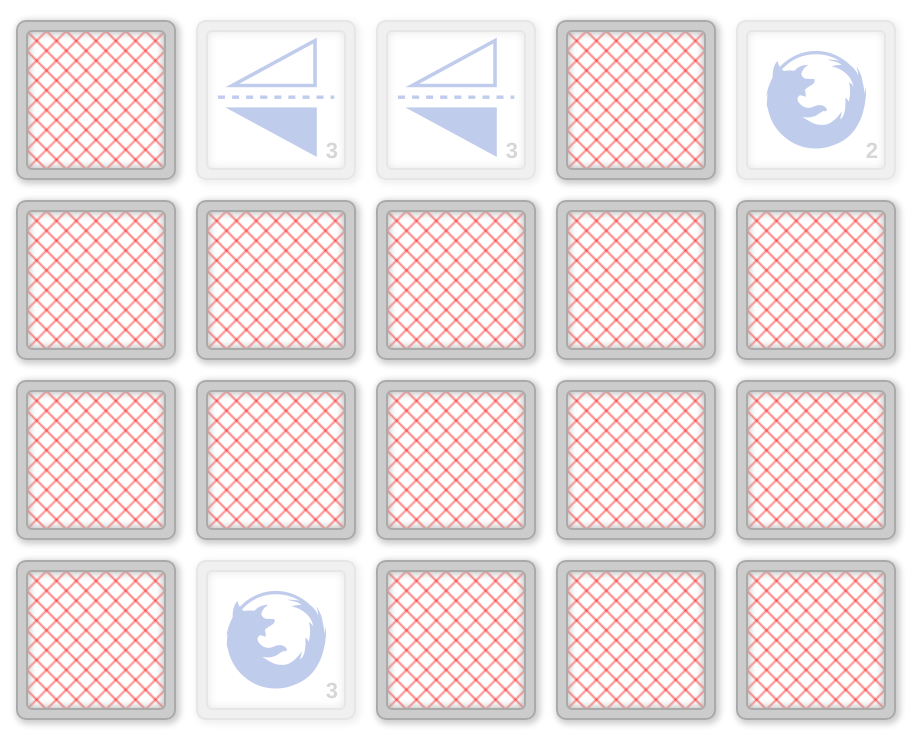# Memory (Concentration)

The Memory Solitaire game consists in flipping pairs of cards laid face down and finding matches.## Description

Memory (also known as Concentration, Match Match, Match Up, and other names) is a solitaire played with a deck of $n$ pairs of matching cards, where the cards of each pair are marked with the same symbol, and different pairs have different symbols. The deck is shuffled and the cards are laid face down (usually in a grid pattern), so that their symbols are hidden. A move of the player consists of sequentially choosing two cards to flip over, i.e., the player flips the first card (and observes its symbol) before choosing and flipping the second one. If the symbols on the two chosen cards match, both cards are removed from the game; otherwise, they are both flipped back over. A game ends when all pairs have been matched (i.e., when no more cards are left).

## Computational complexity

It is easy to see that the number of moves needed in order to complete the game with certainty (assuming perfect memory of the player) is $2n-1$ .

The problem of minimizing the expected number of moves needed to complete the game has been studied in  and .

In  it is shown that, in expectation, every strategy needs at least $1.5 \cdot n - 1$ moves while optimal strategy needs at most $1.75 \cdot n$ moves. The authors of  also conjectured that the optimal strategy has an expected number of moves of $\approx 1.613 \cdot n,$ supporting it with numerical calculations.

If $k$ cards are already known to the player, then at least $\frac{3}{2} \cdot n - \frac{k}{2} -1$ and at most $2n - k$ moves are needed, in expectation .

In  the conjecture of  was proved by showing that the expected number of moves of an optimal strategy is $(3 - 2 \ln 2)n + \frac{7}{8} - 2 \ln 2 \approx 1.613 \cdot n$.

In  the setting where the player does not have perfect memory is analyzed and tight time-space tradeoff bounds are given. Let $T$ be the number of moves and $S$ be the number of bits of memory available to the player. The authors show that if the player can only compare cards for equality, then $ST=\Theta(n^2 \log n)$. If symbols are integers and the player is allowed to perform arbitrary computations on these integers, then $ST^2=\Omega(n^3)$ and it is conjectured that $ST=\tilde\Omega(n^2)$ .K. Foerster and R. Wattenhofer, “The Solitaire Memory Game”, 2013.

 D.J. Velleman and G.S. Warrington, “What to expect in a game of memory”, The American Mathematical Monthly. 2013.A. Chakrabarti and C. Yining. “Time-Space Tradeoffs for the Memory Game”, arXiv preprint, 2017.

Image courtesy of Mark Rolich.

@misc{cog:memory,
author = "{CoG contributors}",
title  = "{Memory (Concentration) --- Complexity of Games}",
year   = "2022",
url    = "https://www.isnphard.com/i/memory/",
note   = "[Online; accessed 2022-06-02]"
}